Next: Running the example Up: Tidal forcing Previous: Initial conditions   Contents

### Boundary conditions

Boundary conditions are given by the first eight tidal constituents as computed by OTIS which can be obtained from
http://www.coas.oregonstate.edu/research/po/research/tide/
Tidal constituent data is read into SUNTANS in the function BoundaryVelocities in the file boundaries.c, and the data is stored in the files specified by the variable TideInput in suntans.dat (the default is tidecomponents.dat). Each processor will require a file with the name tidecomponents.dat.proc, where proc is the processor number. This file contains the tidal constituents for the horizontal velocity components and the free surface height (in cm s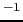and cm) at the locations of the centers of each boundary edge of type 2. These locations are given in the files specified by the variable TideOutput in suntans.dat (the default is tidexy.dat). The TideInput file has the following binary format for each processor:

numtides (1 X int) number of tidal constituents.
numboundaryedges (1 X int) number of boundary edges.
omegas (numtides X REAL) frequencies of tidal components.
u_amp (numtides X REAL) amplitude of easting velocity at location 0.
u_phase (numtides X REAL) phase of easting velocity at location 0.
v_amp (numtides X REAL) amplitude of northing velocity at location 0.
v_phase (numtides X REAL) phase of northing velocity at location 0.
h_amp (numtides X REAL) amplitude of free surface at location 0.
h_phase (numtides X REAL) phase of free surface at location 0.
u_amp (numtides X REAL) amplitude of easting velocity at location 1.
u_phase (numtides X REAL) phase of easting velocity at location 1.
v_amp (numtides X REAL) amplitude of northing velocity at location 1.
v_phase (numtides X REAL) phase of northing velocity at location 1.
h_amp (numtides X REAL) amplitude of free surface at location 1.
h_phase (numtides X REAL) phase of free surface at location 1.
...
u_amp[numboundaryedges-1] (numtides X REAL) amplitude of easting velocity at last location.
u_phase[numboundaryedges-1] (numtides X REAL) phase of easting velocity at last location.
v_amp[numboundaryedges-1] (numtides X REAL) amplitude of northing velocity at last location.
v_phase[numboundaryedges-1] (numtides X REAL) phase of northing velocity at last location.
h_amp[numboundaryedges-1] (numtides X REAL) amplitude of free surface at last location.
h_phase[numboundaryedges-1] (numtides X REAL) phase of free surface at last location

The user can create this file or it can be created using the suntides matlab package, (located in suntans/mfiles/suntides) which is an adaptation of the tidegui package created by Brian Dushaw1. The suntides.m file reads in the x-y locations of the boundary points for each processor and outputs the associated tidal components in binary form. The matlab script tides.m in the tides example directory performs this action. The x-y location data files are created by running SUNTANS, which will complain if no tidecomponents.dat.proc files are found in the data directory, i.e the directory specified in the command line call in suntans with --datadir=. In the present example, this directory is given by data. Upon running the test, SUNTANS will complain with
Error opening data/tidecomponents.dat.0!
Writing x-y boundary locations to data/tidexy.dat.0 instead.
Error opening data/tidecomponents.dat.1!
Writing x-y boundary locations to data/tidexy.dat.1 instead.

This indicates that the tidal component data files were missing, so SUNTANS writes the locations of the markers of type 2 (the boundary edges) to the tidexy.dat.proc files. Once these files have been created, the tides.m script can be run to create the tidecomponents.dat.proc files. This can be run from the command line in unix with matlab < tides.m.

In order to prevent transient oscillations associated with the impulsive starting of the tidal boundary conditions, it is always a good idea to spin-up the boundary conditions over a day or so with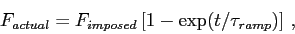so that the forcing approaches the imposed forcing over a time scale of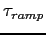. This is implemented in the file boundaries.c in the function BoundaryVelocities, where the tidal data is input. For example, the u-velocity component is imposed with the line
phys->boundary_u[jind][k]=u*(1-exp(-prop->rtime/prop->thetaramptime))/100.0;

Note that the value of u is multiplied by the ramping function as well as divided by 100.0 in order to convert from cm sto m s. The ramping function ramps up over a time scale given by the variable thetaramptime, which is defined as 86400 (one day in seconds) in suntans.dat. This variable also implies more damping during this initial transient period by ramping the value of theta in the simulation from 1 (fully implicit barotropic time-stepping) to the value specified in suntans.dat by the variable theta. Note that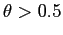for stability, and the value of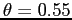is typically used. In the above expression for the damping transient, for the time, the variable rtime is used, which represents the time, in seconds, from the beginning of the simulation, which starts at rtime=0. Using the suntides example, all tidal phases are with reference to the beginning of the year specified when running the suntides.m script. For the present example, the year is given by the line year = 2006 in setup_tides.m. In order to start the simulation from a specific day in 2006, a time offset is specified in suntans.dat as the variable toffSet, which is in days. This offset is read into boundaries.c and converted to seconds with the line
toffSet = MPI_GetValue(DATAFILE,"toffSet","BoundaryVelocities",myproc)*secondsPerDay;Next: Running the example Up: Tidal forcing Previous: Initial conditions   Contents
2014-08-06